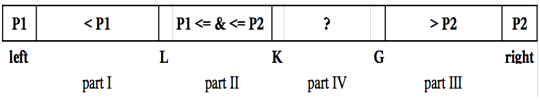### Quicksort 2.0

The new Quicksort algorithm uses partitioning a source array T[ ] a, where T is primitive type […], to three parts defined by two pivot elements P1 and P2 (and therefore, there are three pointers L, K, G, and left and right […]) shown [below]:It is proved that for the Dual-Pivot Quicksort the average number of comparisons is 2*n*ln(n), the average number of swaps is 0.8*n*ln(n), whereas classical Quicksort algorithm has 2*n*ln(n) and 1*n*ln(n) respectively. (Vladimir Yaroslavskiy) [paper, link, Thanks John.]